# LINQ Single and SingleOrDefault Methods

## LINQ Single and SingleOrDefault Methods in C#

In this article, I will discuss the LINQ Single and SingleOrDefault Methods in C# with Examples using Method and Query Syntax. Please read our previous article, discussing the LINQ Last and LastOrDefault Methods in C# with Examples. In LINQ, the Single and SingleOrDefault methods are used to return a single, specific element from a sequence.

#### LINQ Single Method in C#:

The LINQ Single Method returns a single element from a data source or, you can say, from a sequence. It is used when you expect the sequence to contain exactly one element that satisfies a specified condition. Two overloaded versions are available for this LINQ Single Method, shown in the image below.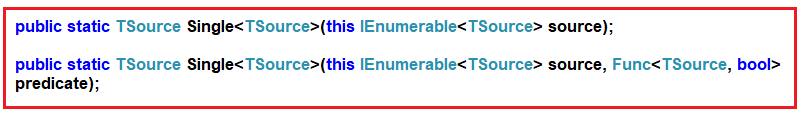As you can see, the first overloaded version of the Single method does not take any parameter. This method returns the only element from a sequence. In this case, if the data source is empty or if the data source contains more than one element, then it throws an exception.

On the other hand, the second overloaded version of the Single method takes one predicate as a parameter, and using this predicate, we can specify a condition. This method returns the only element from the sequence that satisfies the given condition. In this case, the method will throw an exception when the following conditions are true.

1. If the data source is empty.
2. When the given condition does not satisfy any element in the sequence.
3. If the given condition satisfies more than one element.
##### Example to Understand LINQ Single Method in C#

Let us see an example of understanding LINQ Single Method in C# using Method and Query Syntax. In the below example, we have created one data source which contains only one element. Now, when we apply the Single method to this data source, it will return the only element present in the data source.

```using System.Linq;
using System;
using System.Collections.Generic;
namespace LINQSingleMethodDemo
{
class Program
{
static void Main(string[] args)
{
//Sequence contains one element
List<int> numbers = new List<int>() { 10 };

//Fetching the Only Element from the Sequenece using Method Syntax
int numberMS = numbers.Single();

//Fetching the Only Element from the Sequenece using Query Syntax
int numberQS = (from num in numbers
select num).Single();

//Printing the Returned element by Single Method
Console.WriteLine(numberQS);
}
}
}
```

Output: 10

##### LINQ Single Method with Empty Data Source

Let us see what happens when we call the LINQ Single Method on an Empty Data Source. In the following example, the data source is empty. When we apply the LINQ Single method on the empty data source, it will throw System.InvalidOperationException: ‘Sequence contains no elements’ exception.

```using System.Linq;
using System;
using System.Collections.Generic;
namespace LINQSingleMethodDemo
{
class Program
{
static void Main(string[] args)
{
//Sequence contains no element i.e. Empty Data Source
List<int> numbers = new List<int>();

//Fetching the Only Element from the Sequenece using Method Syntax
int numberMS = numbers.Single();

//Fetching the Only Element from the Sequenece using Query Syntax
int numberQS = (from num in numbers
select num).Single();

//Printing the Returned element by Single Method
Console.WriteLine(numberQS);
}
}
}
```

Now run the application, and you will get the following exception. Here, we get the Invalid Operation Exception, which clearly shows why the sequence contains no elements.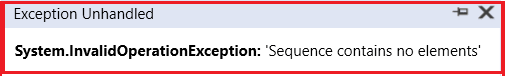##### What happens when the Sequence Contains more than one element?

If the Sequence contains more than one element and we apply the Single method to that sequence, we will get the System.InvalidOperationException: ‘Sequence contains more than one element’ Exception. In the following example, we applied the Single method to a data source that contains more than one element.

```using System.Linq;
using System;
using System.Collections.Generic;
namespace LINQSingleMethodDemo
{
class Program
{
static void Main(string[] args)
{
//Sequence contains more than one element
List<int> numbers = new List<int>() { 10, 20, 30 }; ;

//Fetching the Only Element from the Sequenece using Method Syntax
int numberMS = numbers.Single();

//Fetching the Only Element from the Sequenece using Query Syntax
int numberQS = (from num in numbers
select num).Single();

//Printing the Returned element by Single Method
Console.WriteLine(numberQS);
}
}
}
```

When you run the above application, you will get the following exception. This is because now the sequence contains more than one element, clearly told by the following exception message.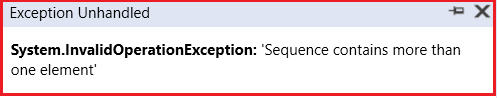##### Using the Second Overloaded Version of the Single Method, which takes the Predicate as a Parameter:

Suppose the sequence contains more than one element, and we need to fetch a single element based on some condition. In that case, we need to use the second overloaded version of the LINQ Single method, which takes one predicate as a parameter. Let us understand this with an example. In the following example, we use the Single method, which takes one predicate as a parameter. We specify our condition using that predicate, which will return only one element from the sequence. Here, we are returning the element which is equal to 20.

```using System.Linq;
using System;
using System.Collections.Generic;
namespace LINQSingleMethodDemo
{
class Program
{
static void Main(string[] args)
{
//Sequence contains more than one element
List<int> numbers = new List<int>() { 10, 20, 30 }; ;

//Fetching the Only Element from the Sequenece using Method Syntax
//Where the Element is 20
int numberMS = numbers.Single(num => num == 20);

//Fetching the Only Element from the Sequenece using Query Syntax
//Where the Element is 20
int numberQS = (from num in numbers
select num).Single(num => num == 20);

//Printing the Returned element by Single Method
Console.WriteLine(numberQS);
}
}
}
```

Output: 20

Here, we will get the output as expected, as the specified condition returns a single element from the sequence.

##### What Happens if the Condition Specified in the Single Method Returns More than One Element?

If the Condition Specified in the Single Method Returns more than One Element, we will get System.InvalidOperationException: ‘Sequence contains more than one matching element’ exception. Let us understand this with an example. In the below example, the specified condition returns more than one element, and hence, we will get an exception saying the sequence contains more than one matching element.

```using System.Linq;
using System;
using System.Collections.Generic;
namespace LINQSingleMethodDemo
{
class Program
{
static void Main(string[] args)
{
//Sequence contains more than one element
List<int> numbers = new List<int>() { 10, 20, 30 }; ;

//Fetching the Only Element from the Sequenece using Method Syntax
//Where the Element > 10
int numberMS = numbers.Single(num => num > 10);

//Fetching the Only Element from the Sequenece using Query Syntax
//Where the Element > 10
int numberQS = (from num in numbers
select num).Single(num => num > 10);

//Printing the Returned element by Single Method
Console.WriteLine(numberQS);
}
}
}
```

Now run the application, and you will get the following exception and clearly tell us that Sequence contains more than one matching element.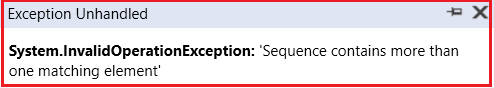##### What Happens if the Condition Specified in the Single Method does not return any data?

If the Condition Specified in the Single Method returns no data, we will get System.InvalidOperationException: ‘Sequence contains no matching element’ exception. Let us understand this with an example. In the below example, the specified condition does not return any data, and hence, we will get an exception saying the sequence contains no matching elements.

```using System.Linq;
using System;
using System.Collections.Generic;
namespace LINQSingleMethodDemo
{
class Program
{
static void Main(string[] args)
{
//Sequence contains more than one element
List<int> numbers = new List<int>() { 10, 20, 30 }; ;

//Fetching the Only Element from the Sequenece using Method Syntax
//Where the Element < 10
int numberMS = numbers.Single(num => num < 10);

//Fetching the Only Element from the Sequenece using Query Syntax
//Where the Element < 10
int numberQS = (from num in numbers
select num).Single(num => num < 10);

//Printing the Returned element by Single Method
Console.WriteLine(numberQS);
}
}
}
```

When you run the application, you will get the following exception.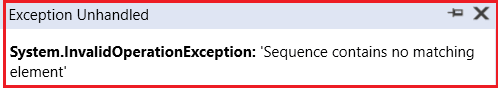##### When to use the LINQ SingleOrDefault Method in C#?

If you don’t want to throw an exception when the sequence is empty or if the specified condition does not return an element from a sequence, then you need to use the LINQ SingleOrDefault method. The LINQ SingleOrDefault method is very similar to the LINQ Single method, except that this method will not throw an exception when the sequence is empty or no element satisfies the given condition, it returns a default value (e.g., null for reference types, 0 for int, etc.) if the sequence is empty.

This method returns the only element of a sequence that satisfies a specified condition or a default value if no such element exists; this method throws an exception if more than one element satisfies the condition.

The most important point you need to remember is that, like the Single method, the SingleOrDefault method still throws an exception when the sequence contains more than one matching element for the given condition. Like the Single Method, two overloaded versions are available for this method in LINQ. They are as follows.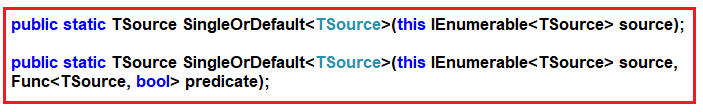As you can see, the first overloaded version of this method does not take any parameters. It simply returns the only element from the sequence. If the sequence is empty, then it returns the default value without throwing an exception. If the sequence contains multiple elements, it will throw an exception.

We can specify a condition using the second overloaded version of this method. If the specified condition does not return any data, it will not throw an exception. Instead, it returns the default value. But if the specified condition matches multiple data in the data source, it will throw an exception.

##### Example to Understand LINQ SingleOrDefault Method in C#

Let us see an example of the LINQ SingleOrDefault method in C# with both Method and Query Syntax. In the following example, the specified condition does not match any elements in the sequence. So, in this case, the SingleOrDefault method will return 0 as 0 is the default value for the integer data type.

```using System.Linq;
using System;
using System.Collections.Generic;
namespace LINQDemo
{
class Program
{
static void Main(string[] args)
{
List<int> numbers = new List<int>() {10, 20, 30 };
int number = numbers.SingleOrDefault(num => num < 10);
Console.WriteLine(number);
}
}
}
```

Output: 0

In the following example, the sequence is empty. But as we use the SingleOrDefault method, we will not get any exception when we run the below example; instead, we will get the default value 0.

```using System.Linq;
using System;
using System.Collections.Generic;
namespace LINQSingleMethodDemo
{
class Program
{
static void Main(string[] args)
{
//Sequence contains no element
List<int> numbers = new List<int>();

//Fetching the Only Element from the Sequenece using Method Syntax
//Where the Element < 10
int numberMS = numbers.SingleOrDefault(num => num < 10);

//Fetching the Only Element from the Sequenece using Query Syntax
//Where the Element < 10
int numberQS = (from num in numbers
select num).SingleOrDefault(num => num < 10);

//Printing the Returned element by Single Method
Console.WriteLine(numberQS);
}
}
}```

Output: 0

##### What happens when the specified condition returns more than one element?

We will get an exception if the specified condition matches with more than one element. In the following example, we will get an exception. This is because the specified condition matches more than one element.

```using System.Linq;
using System;
using System.Collections.Generic;
namespace LINQSingleMethodDemo
{
class Program
{
static void Main(string[] args)
{
//Sequence contains
List<int> numbers = new List<int>() { 10, 20, 30 };

//Fetching the Only Element from the Sequenece using Method Syntax
//Where the Element > 10
int numberMS = numbers.SingleOrDefault(num => num > 10);

//Fetching the Only Element from the Sequenece using Query Syntax
//Where the Element > 10
int numberQS = (from num in numbers
select num).SingleOrDefault(num => num > 10);

//Printing the Returned element by Single Method
Console.WriteLine(numberQS);
}
}
}```

When you run the above code, you will get the following exception.##### Key points to consider when using these methods:
• Use Single when you expect exactly one element to match the condition, and an exception is appropriate if that condition is not met or if there are multiple matches.
• Use SingleOrDefault when you want to handle cases where no matching element is found or when multiple matching elements are encountered without throwing exceptions.
• Always be cautious when using Single and SingleOrDefault to ensure that your assumptions about the number of matching elements are accurate to avoid unexpected exceptions or results.
• You can use these methods with various LINQ query operators and in conjunction with other LINQ methods to filter and retrieve specific elements from collections based on your criteria.
###### What is the Difference Between LINQ Single and SingleOrDefault Methods in C#?

The Single and SingleOrDefault methods in LINQ are used to return a single element from a sequence, but they handle the case of an empty sequence differently:

###### Single:
• Returns the only element of a sequence and throws an exception if the sequence is empty or if there is more than one element.
• Use Single when confident that the sequence should contain exactly one element. If the sequence does not meet this expectation, an exception is appropriate.
###### SingleOrDefault:
• Returns the only sequence element or default value if the sequence is empty. However, if there is more than one element in the sequence, it will still throw an exception.
• Use SingleOrDefault when you expect the sequence to have at most one element. If the sequence is empty, you want to handle it gracefully by receiving a default value instead of an exception.

So, choose Single when the absence of exactly one element is considered an error in the context of your application. Choose SingleOrDefault when zero elements are acceptable, but more than one element is still considered an error.

In the next article, I will discuss the Linq LINQ DefaultIfEmpty Method in C# with Examples. In this article, I try to explain the LINQ Single and SingleOrDefault Methods in C# with Examples. I hope you understand the need and use of LINQ Single and SingleOrDefault Methods.